# Numeric Functions in Oracle

## Numeric Functions in Oracle with Examples

In this article, I am going to discuss Numeric Functions in Oracle with Examples. Please read our previous article where we discussed Functions in Oracle. At the end of this article, you will understand the following oracle Numeric Functions with examples.

1. ABS() Function
2. CEIL() Function
3. FLOOR() Function
4. MOD() Function
5. POWER() Function
6. ROUND() Function
7. TRUNC() Function
##### 1) ABS() Function in Oracle:

This Numeric Function in Oracle is used to convert (-VE) value into (+VE) value. That means the ABS function in Oracle returns the absolute value of n. This function takes as an argument any numeric datatype or any non-numeric data type that can be implicitly converted to a numeric datatype. The function returns the same datatype as the numeric datatype of the argument. The syntax to use the ABS function is given below.

Syntax: ABS(number)

Example:
SELECT ABS(-12) FROM DUAL;
Output: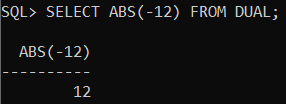##### 2) CEIL() Function In Oracle:

This Numeric Function in Oracle is used to return a value that is greater than or equal to the given value. That means the CEIL function in oracle returns the smallest integer greater than or equal to n. This function takes as an argument any numeric datatype or any non-numeric data type that can be implicitly converted to a numeric datatype. The function returns the same datatype as the numeric datatype of the argument. The syntax to use CEIL function is given below.

Syntax: CEIL(NUMBER)

Example:
SELECT CEIL(9.0) FROM DUAL;
Output: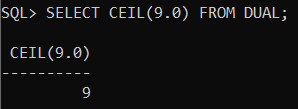Example:
SELECT CEIL(9.3) FROM DUAL;
Output: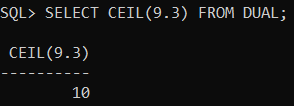##### 3) FLOOR() Function in Oracle:

This numeric function is used to return the largest integer equal to or less than n. This function takes as an argument any numeric datatype or any non-numeric data type that can be implicitly converted to a numeric datatype. The function returns the same datatype as the numeric datatype of the argument. The syntax to use the FLOOR function is given below.

Syntax: FLOOR(NUMBER)

Example:
SELECT FLOOR(9.0) FROM DUAL;
Output: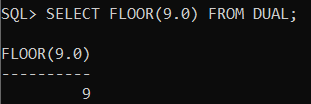Example:
SELECT FLOOR(9.8) FROM DUAL;
Output: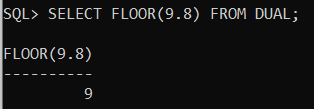##### 4) MOD() Function in Oracle:

The Oracle MOD() function is used to return the remainder value. That means this Numeric Function in Oracle is used to return the remainder of a dividend divided by a divisor. This function takes as arguments any numeric datatype or any non-numeric data type that can be implicitly converted to a numeric datatype. Oracle determines the argument with the highest numeric precedence, implicitly converts the remaining arguments to that datatype and returns that datatype. The syntax to use the MOD function is given below.

Syntax: MOD(m, n)

Example:
SELECT MOD(10,2) FROM DUAL;
Output: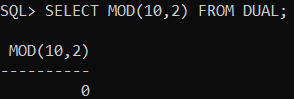Example:
SELECT MOD(15,4) FROM DUAL;
Output: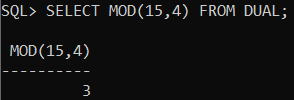##### 5) POWER() Function in Oracle:

This numeric function is used to return the power of a given expression. The POWER Function in Oracle returns n2 raised to the n1 power. The base n2 and the exponent n1 can be any numbers, but if n2 is negative, then n1 must be an integer. This POWER function takes as arguments any numeric datatype or any non-numeric data type that can be implicitly converted to a numeric datatype. If any argument is BINARY_FLOAT or BINARY_DOUBLE, then the function returns BINARY_DOUBLE. Otherwise, the function returns NUMBER. The syntax to use the POWER function is given below.

Syntax: POWER(m, n)

Example:
SELECT POWER(2, 3) FROM DUAL;
Output: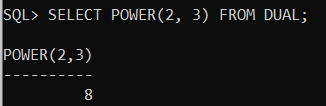##### 6) ROUND() Function in Oracle:

This numeric function returns the nearest value of the given expression. The ROUND function in oracle returns n rounded to integer places to the right of the decimal point. If you omit integer, then n is rounded to 0 places. The argument integer can be negative to round off digits left of the decimal point. The syntax to use the ROUND function is given below.

Syntax: ROUND(NUMBER,[DECIMAL PLACES])

Example: The following example rounds a number to one decimal point
SELECT ROUND(15.253, 1) FROM DUAL;
Output: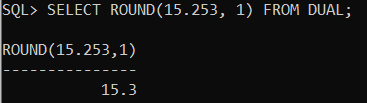Example: The following example rounds a number one digit to the left of the decimal point.
SELECT ROUND(15.253,-1) FROM DUAL;
Output: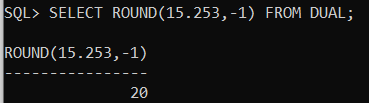Example: The following examples illustrate the difference between rounding NUMBER and floating-point number values. NUMBER values are rounded up (for positive values), whereas floating-point numbers are rounded toward the nearest even value.
SELECT ROUND(2.5), ROUND(3.5) FROM DUAL;
Output: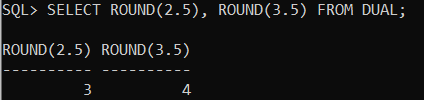Example:
SELECT ROUND(2.5f), ROUND(3.5f) FROM DUAL;
Output: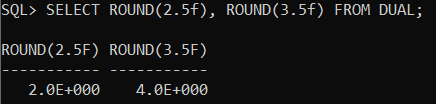Example: The following example rounds a number to two decimal point
SELECT ROUND(32.456,2) FROM DUAL;
Output: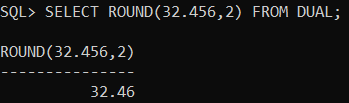##### 7) TRUNC() Function in Oracle:

The TRUNC (number) function in Oracle is used to return n1 truncated to n2 decimal places. If n2 is omitted, then n1 is truncated to 0 places. n2 can be negative to truncate (make zero) n2 digits left of the decimal point. This function takes as an argument any numeric datatype or any non-numeric data type that can be implicitly converted to a numeric datatype. If you omit n2, then the function returns the same datatype as the numeric datatype of the argument. If you include n2, then the function returns NUMBER. The syntax to use the TRUNC (number) function in Oracle is given below.

Syntax: TRUNC (NUMBER, DECIMAL PLACES)

Example:
SELECT TRUNC(5.50) FROM DUAL;
Output: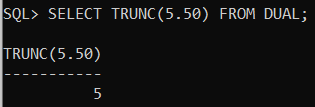Example:
SELECT TRUNC(32.456,2) FROM DUAL;
Output: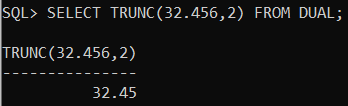Example:
SELECT TRUNC(32.456,-1) FROM DUAL;
Output: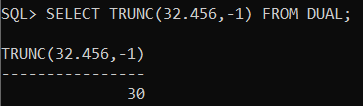In the next article, I am going to discuss String Functions in Oracle with Examples. Here, in this article, I try to explain Numeric Functions in Oracle with Examples and I hope you enjoy this Numeric Functions in Oracle with Examples article.